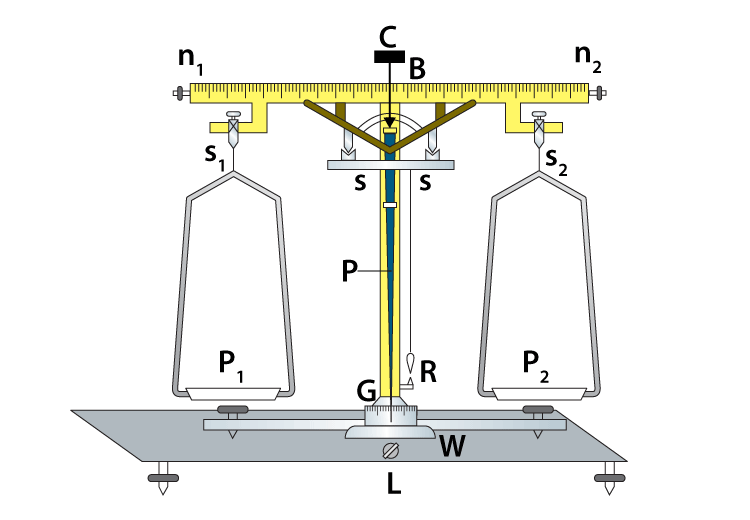# To Determine The Mass Of Two Different Objects Using A Beam Balance

A beam balance is a device that is used to determine the mass of a body under gravitation, i.e., weight. It consists of a beam at the centre supported by an agate knife resting on a support moving inside a vertical pillar. In this class 11 physics experiment, we will be discussing how to determine the mass of two different objects using a beam balance.

## Aim

To determine the mass of two different objects using beam balance.

## Apparatus/ Materials Required

• Weight Box
• Beam Balance
• Forceps
• Beam Balance of different masses

### Diagram## Theory

A physical balance determines the gravitational mass of a body by making use of the principle of moments.

m1g × a1 = m2g × a2

m1a1 = m2a2

A body with a gravitational mass m1 is placed in the left pan and a standard weight of m2 is placed in the right pan to keep the beam horizontal for a beam balance, a1 = a2, then m1 = m2.

In other words, the gravitational mass of the body in the left pan = the gravitational mass in the right pan.

## Procedure

1. Adjust the physical beam. When the beam is in the raised position, the pointer will stay at rest and coincide with the zero division or moves to and fro over the scalp, equally over the zero division.
2. When the beam is in the rest position, put any one of the objects in the left pan. Now put some standard weights with half of the forceps from the weight box. Shut the front glass door in order to avoid the air current disturbance. Raise the beam with the help of the handle and make sure that the beam is horizontal and the pointer moves to and fro equally on both sides of zero division. If not, put or remove some weight to get the correct horizontal position of the beam and pointer.
3. Repeat step 2 for the second object.

## Observation

 S.No Body Weight in x (g) Weight in y (mg) Total weight (x+y) g 1 | ……… ……… ……… 2 || ……… ……… ………

## Result

The gravitational mass of the bodies are:

(i) mass of the body m1 is _____ g

(ii) mass of the body m2 is _____ g

## Viva Voce

1. What is mass?

Answer: The quantity of matter possessed by a body is known as mass.

2. What are the units of mass?

Answer: The SI unit of mass is kilograms while the C.G.S unit of mass is grams.

3. Define weight.

Answer: The force by which the body is attracted by the earth towards its centre is known as weight.

4. Describe phenomena in which both inertial mass and gravitational mass take part?

Answer: In the simple harmonic motion of the bob of the pendulum both inertial and gravitational masses of the bob play their part.

5. Explain the working principle of the spring balance.

Answer: The spring balance works on the principle of Hooke’s law. It works on the fact that when a body is suspended from a vertical spring, the body produces an extension in the length of the spring proportional to its weight.

6. What is true balance?

Answer: True balance is a balance that has arms of equal lengths and pans of equal weight.

7. What is the astronomical unit of mass?

Answer: The astronomical unit of mass is solar mass.

Stay tuned with BYJU’S to get the latest notification on CBSE along with CBSE syllabus, sample papers, marking scheme, and more.

## What does a weighing machine measure?-->
Test your knowledge on Beam balance experiment